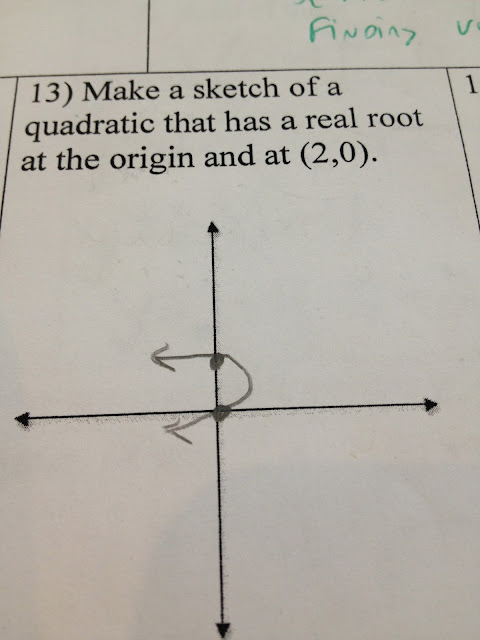## Tuesday, January 1, 2013

### Algebra II (4) Assessment Reflection #6

January 1, 2013

The average was 74% for my two classes. This was the toughest quiz to date. The quiz came immediately before vacation and was the first time students had to use the Quadratic formula. In class we are going to look closely at student work on this post.

All comments are for the picture immediately below.

This graph is going the wrong way...This is a nice job especially since the graph is upside down.

Below, where is the origin?

When writing an equation of a quadratic we must know which equation (vertex or intercept form) is most appropriate to start with.  Here, the student used vertex form incorrectly.  This problem gives you the x intercepts so intercept form is the best first step.
Below, same mistake.  This equation could never be right since there is no "x" value.

Now lets look at some problems that involve simplifying the Quadratic Formula. Here the student made a common mistake of not making the part under the radical a negative 108.  Also, the square root of 108 can be simplified.

Next this student used "r" in the quadratic formula, when all of the coefficients were already known.   The work should have been only numbers.

Below, the 6 in the denominator was divided evenly into the part under the radical.

Below, can you spot the small simplifying mistake?

Below, the same type of error was made like the problem above.
Finally some small errors to be mindful of...

Below is the assessment data. Raw % and % Correct when partial credit is factored in.  Quadratics is a major part of our midterm exam and we'll be spending time before midterms reinforcing this unit [and the topics that came before like equations of lines and systems].

My Best,

Patrick Morrissey